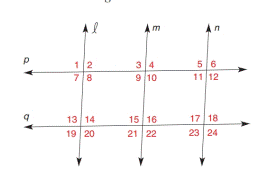Chapter 2.3, Problem 15E### Elementary Geometry for College St...

6th Edition
Daniel C. Alexander + 1 other
ISBN: 9781285195698

#### Solutions

Chapter
Section### Elementary Geometry for College St...

6th Edition
Daniel C. Alexander + 1 other
ISBN: 9781285195698
Textbook Problem
6 views

# In Exercise 7 to 16, name the lines (if any) that must be parallel under the given conditions.m ∠ 8 = 110 ° , p ∥ q , and m ∠ 18 = 70 °

To determine

To find:

The parallel lines.

Explanation

Given:

The given statement is,

m8=110°, pq, and m18=70°.

Approach:

(1) If two lines are cut by a transversal so that two corresponding angles are congruent, then these lines are parallel.

(2) If two parallel lines are cut by a transversal, then the pairs of interior angles on the same side of the transversal are supplementary.

Calculation:

The given statement is,

m8=110°, pq, and m18=70°.

8 and 14 are interior angles on the same side of transversal which cut two parallel lines p and q.

So,

m8+m14=180°

Substitute 110° for m8 in the above equation

### Still sussing out bartleby?

Check out a sample textbook solution.

See a sample solution

#### The Solution to Your Study Problems

Bartleby provides explanations to thousands of textbook problems written by our experts, many with advanced degrees!

Get Started

#### What are the two requirements for a random sample?

Essentials of Statistics for The Behavioral Sciences (MindTap Course List)

#### True or False: f(x) = 4x2 8x + 1 is increasing on (0, 1).

Study Guide for Stewart's Single Variable Calculus: Early Transcendentals, 8th

#### Suppose N = 15 and r = 4. What is the probability of x = 3 for n = 10?

Statistics for Business & Economics, Revised (MindTap Course List)